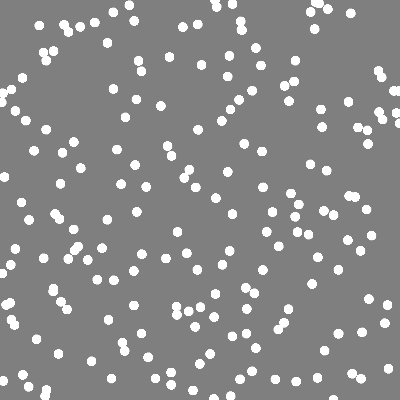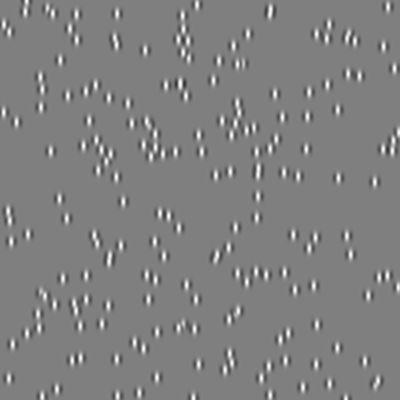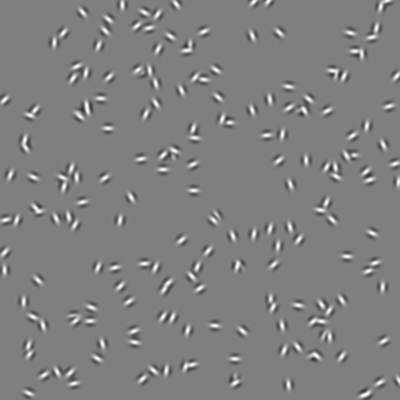# Drawing—dots

Objectives

• Be able to create and manipulate stimuli formed from a number of individual elements.

Patterns formed from a number of small elements (“dots”, typically circles, Gaussians, or squares) are versatile stimuli that are frequently used in vision research. In psychopy, we typically create dot stimuli using `ElementArrayStim`.

The key point about `ElementArrayStim` is that many of its parameters accept a list, rather than only a single value. This allows us to conveniently create a stimulus from many individual elements. The `xys` is a critical parameter that specifies the position of each element. For example, we can draw a set of randomly-positioned dots by:

```import random

import psychopy.visual
import psychopy.event

win = psychopy.visual.Window(
size=[400, 400],
units="pix",
fullscr=False
)

n_dots = 200

dot_xys = []

for dot in range(n_dots):

dot_x = random.uniform(-200, 200)
dot_y = random.uniform(-200, 200)

dot_xys.append([dot_x, dot_y])

dot_stim = psychopy.visual.ElementArrayStim(
win=win,
units="pix",
nElements=n_dots,
elementTex=None,
xys=dot_xys,
sizes=10
)

dot_stim.draw()

win.flip()

psychopy.event.waitKeys()

win.close()
```There are a few things to note about the code above:

• We specify `xys` as a list of lists; the “outer” list has a number of elements that matches the number of dots. Each element is itself a list, with two elements—the x and y location of that particular dot.
• Note the use of `elementTex` here. By setting it to the special Python value `None`, we are telling psychopy that we want the “texture” of each dot to just be uniform white. Then, by setting `elementMask` to `"circle"`, we are able to define the shape of each individual dot.
• Notice how the size of the dots is specified by the parameter `sizes`, rather than the typical `size`. That means that we could provide a list with a length corresponding to the number of dots, with each element of the list specifying the size of that particular dot. By just giving a single number here, we are telling psychopy to use this value for all of the dots.

The `ElementArrayStim` doesn’t just have to be used for shape-based dots, though. By setting the `elementTex` and `elementMask` parameters, we have great flexibility in what comprises our stimulus. For example, we could use lots of little gratings:

```import random

import psychopy.visual
import psychopy.event

win = psychopy.visual.Window(
size=[400, 400],
units="pix",
fullscr=False
)

n_dots = 200

dot_xys = []

for dot in range(n_dots):

dot_x = random.uniform(-200, 200)
dot_y = random.uniform(-200, 200)

dot_xys.append([dot_x, dot_y])

dot_stim = psychopy.visual.ElementArrayStim(
win=win,
units="pix",
nElements=n_dots,
elementTex="sin",
sfs=5.0 / 2.5,
xys=dot_xys,
sizes=20
)

dot_stim.draw()

win.flip()

psychopy.event.waitKeys()

win.close()
```Tip

In `ElementArrayStim`, the `sfs` parameter when `units="pix"` is the number of cycles per element, rather than the number of cycles per pixel.

Perhaps with random orientations:

```import random

import psychopy.visual
import psychopy.event

win = psychopy.visual.Window(
size=[400, 400],
units="pix",
fullscr=False
)

n_dots = 200

dot_xys = []
dot_oris = []

for dot in range(n_dots):

dot_x = random.uniform(-200, 200)
dot_y = random.uniform(-200, 200)

dot_xys.append([dot_x, dot_y])

dot_oris.append(random.uniform(0, 180))

dot_stim = psychopy.visual.ElementArrayStim(
win=win,
units="pix",
nElements=n_dots,
elementTex="sin",
sfs=5.0 / 2.5,
xys=dot_xys,
sizes=20,
oris=dot_oris
)

dot_stim.draw()

win.flip()

psychopy.event.waitKeys()

win.close()
```